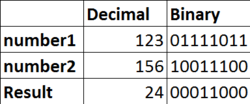# Documentation/Calc Functions/BITAND

Other languages:
dansk • ‎English

BITAND

Mathematical

## Summary:

Returns a bitwise logical "and" of the parameters. Both values at the same position should be 1 in the binary representation of the parameter numbers, to get the output as 1 at that position.Note: This function is available since LibreOfficeDev 3.5.

## Syntax:

BITAND(Number 1; Number 2)

## Returns:

BITAND returns a positive integer.

## Arguments:

Number 1 and Number 2 are positive integers less than 2 ^ 48 (281,474,976,710,656).

• If either argument is less than 0, BITAND returns the Err:502.
• If either argument is a decimal number then its floor value is considered.
• If either argument is a non-integer or is greater than (2^48)-1, BITAND returns the Err:502.

None

## Examples:

Formula Description Returns
=BITAND(6;10) 6 in binary is 0110 and 10 in binary is 1010 so
`(0110 & 1010 = 0010)`
2 (0010)
=BITAND(123;156) 123 in binary is 01111011 and 156 in binary is 10011100 so
`(01111011 & 10011100)`
24 (00011000)
=BITAND(6.123;10) floor value of 6.123 is taken so 6 in binary is 0110 and 10 in binary is 1010 so
`(0110 & 1010 = 0010)`
2 (0010)
=BITAND(123; 2^48 + 5) number 2 argument is greater than 2^48 hence it will return an error Err:502
=BITAND(123; -5) number 2 argument is negative hence it will return an error Err:502BITAND example showing that if both number have 1 at a position then then the function will at that position.

AND

BITOR

BITXOR

## ODF standard:

Section 6.6.2, part 2

BITAND